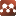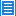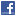Relativity and CosmologyModified General Relativity

Authors: Gary Nash

A modified Einstein equation of general relativity is obtained by using the principle of least action, a decomposition of symmetric tensors on a time oriented Lorentzian manifold, and a fundamental postulate of general relativity. The decomposition introduces a new symmetric tensor $\varPhi_{\alpha\beta}$ which describes the energy-momentum of the gravitational field itself. It completes Einstein's equation and addresses the energy localization problem. The positive part of $\Phi$, the trace of the new tensor with respect to the metric, describes dark energy. The cosmological constant must vanish and is dynamically replaced by $\Phi$. A cyclic universe which developed after the Big Bang is described. The dark energy density provides a natural explanation of why the vacuum energy density is so small, and why it dominates the present epoch of the universe. The negative part of $\Phi$ describes the attractive self-gravitating energy of the gravitational field. $\varPhi_{\alpha\beta}$ introduces two additional terms into the Newtonian radial force equation: the force due to dark energy and the $\frac{1}{r}$ "dark matter" force. When the dark energy force balances the Newtonian force, the flat rotation curves and the baryonic Tully-Fisher relation are obtained. The Newtonian rotation curves for galaxies with no flat orbital curves, and those with rising rotation curves for large radii are described as examples of the flexibility of the orbital rotation curve equation.

Submission history

[v1] 2018-12-06 22:13:04 (removed)
[v2] 2018-12-11 09:59:51
[v3] 2019-03-18 08:08:15
[v4] 2019-04-10 10:41:28